How do we obtain approximation ratio of a real number? Suppose you have a number with several decimal digits like this: 2.14567, what is the best rational approximation of this number? What is the error of this approximation? Try the Decimal to Rational number calculator below.

or

First,

Then, ; Select digit of accuracy = .

Of course, we can always say that 2.14567 = 214567/100000 without any approximation. However, some number like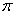may not have the exact decimal number and we can use approximation of continued fraction using this simple algorithm:

## Algorithm:

Suppose we have a decimal number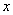At the beginning, we compute term, error and reciprocal of remainder respectively as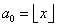,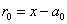,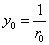Then continued the iteration with iteration formula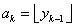,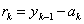,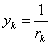, for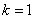to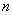Once we get the term of continued fraction, we can use the difference equation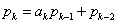,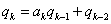to get the ratio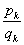The iteration is stop when the absolute error is very small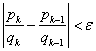,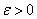and we report the result of one iteration before.

## Example (hand calculation):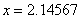, and we set accuracy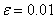we want to get the fraction approximation.

We start with making a continued fraction. We use difference equation with initial condition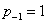,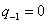,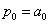,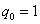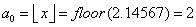,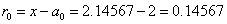,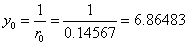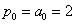,(by definition), then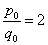with absolute error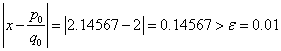, thus we continue the iteration

The first iteration we have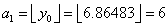,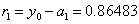,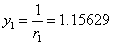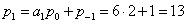,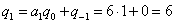, then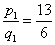with absolute error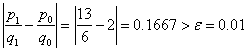, thus we continue the iteration

The second iteration we have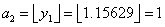,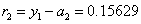,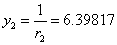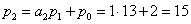,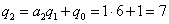, then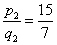with absolute error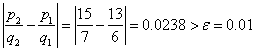, thus we continue the iteration

The third iteration we have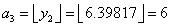,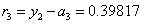,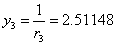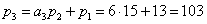,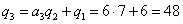, then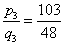with absolute error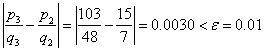, thus we stop the iteration and report the conversion of decimal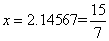with remainder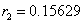## Implementation Code

Visual Basic code for this conversion is given below

`Function Decimal2Fraction(ByVal decNum As Double, ByVal accuracy As Double, _ `
`ByRef nominator, ByRef denominator, ByRef remainder) `
```' Input decNum always between zero to 1

' copyright (c) Kardi Teknomo 2006-2023

' http://people.revoledu.com/kardi```
`Dim a(25) ' I assume the accuracy is lower than 25 digit decimals `
`Dim y(25), r(25) `
`Dim p(25), q(25) `
`Dim error `
`Dim i As Integer, k As Integer `
`Dim ratio As Double, prevRatio As Double `

`For k = 0 To 24 `
`If k = 0 Then `
`a(0) = Int(decNum) ' function Int in VB is equivalent to Floor `
`r(0) = decNum - a(0) `
`If r(0) <> 0 Then y(0) = 1 / r(0) `
`p(0) = a(0) `
`q(0) = 1 `
`ratio = p(0) / q(0) `
`error = Abs(decNum - ratio) `
`Else `
`a(k) = Int(y(k - 1)) ' function Int in VB is equivalent to Floor `
`r(k) = y(k - 1) - a(k) `
`y(k) = 1 / r(k) `
`If k = 1 Then `
`p(1) = a(1) * p(0) + 1 `
`q(1) = a(1) * q(0) `
`Else `
`p(k) = a(k) * p(k - 1) + p(k - 2) `
`q(k) = a(k) * q(k - 1) + q(k - 2) `
`End If `
`ratio = p(k) / q(k) `
`error = Abs(ratio - prevRatio) `
`End If `
`If error < accuracy Then `
`Exit For `
`Else `
`prevRatio = ratio `
`End If `
`Next k `
`' report the results `
`nominator = p(k - 1) `
`denominator = q(k - 1) `
`remainder = r(k - 1) `
`Decimal2Fraction = nominator / denominator `
`End Function `

Try the online interactive program that use the above Decimal to Fraction code so that you can see the code in action.

Alternatively, you may use the program or spreadsheet companion of this tutorial to get conversion of any decimal numbers. For example, try interesting number such as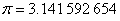and try different accuracy

Preferable reference for this tutorial is

Teknomo, Kardi (2015) Continued Fraction. http://people.revoledu.com/kardi/tutorial/ContinuedFraction/index.html